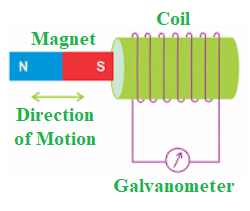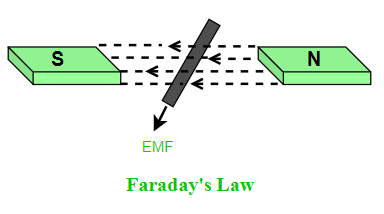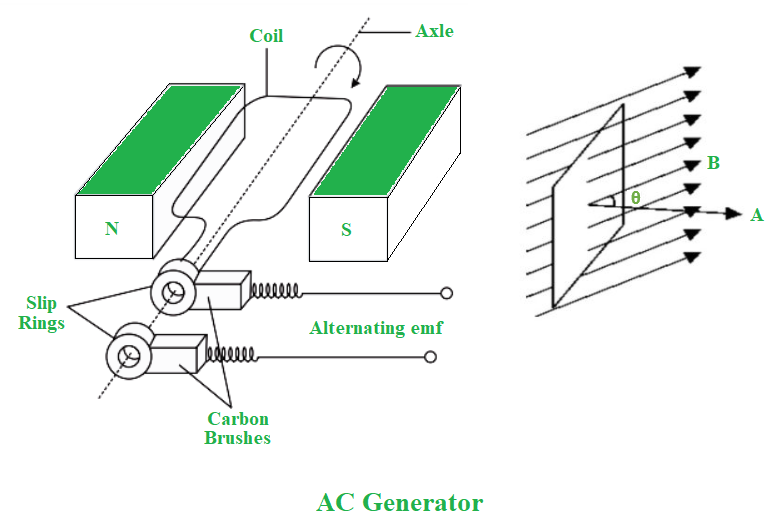GFG App
Open AppBrowser
Continue

# Electromagnetic Induction

Electromagnetic induction, often known as induction, is a process in which a conductor is placed in a certain position and the magnetic field varies or remains stationary as the conductor moves. A voltage or EMF (Electromotive Force) is created across the electrical conductor as a result of this. In 1830, Michael Faraday discovered the Law of Induction. Let’s take a closer look at Electromagnetic Induction.

### Electromagnetic induction

Assume you go cashless when shopping and your parents utilize credit cards. The card is always scanned or swiped by the shopkeeper. The shopkeeper does not photograph or touch the card. However, he swipes/scans it. How does this swiping of the card remove money? This is due to a phenomenon known as ‘Electromagnetic Induction.’

Is it possible for moving things to generate electric currents? How can you tell whether there’s a connection between electricity and magnetism? Consider what life would be like if there were no computers, phones, or electricity. Faraday’s experiments resulted in the development of generators and transformers.The induction of an electromotive force by the passage of a conductor through a magnetic field or by a change in magnetic flux in a magnetic field is known as electromagnetic induction.

This happens when a conductor is placed in a moving magnetic field or when it moves in a fixed magnetic field.

Michael Faraday discovered this electromagnetic induction rule. He put up a leading wire similar to the diagram above, which he linked to a device that measured the voltage across the circuit. The voltage in the circuit is measured when a bar magnet passes through the device. The significance of this is that it is a method of creating electrical energy in a circuit by employing magnetic fields rather than batteries. The principle of electromagnetic induction is used by devices such as generators, transformers, and motors.

### Faraday’s law of Electromagnetic Induction

Faraday gave two laws of electromagnetic induction:

First law: When a conductor is put in a changing magnetic field, an induced emf is produced, and if the conductor is closed, an induced current flows through it.Second Law: The magnitude of the induced EMF is equal to the flux change rate.

Faraday discovered that various elements impact the generation of voltage through his experiment. They are as follows:

• Number of Coils: The induced voltage is proportional to the wire’s number of turns/coils. The more turns there are, the more voltage is created.
• Changing Magnetic Field: The induced voltage is affected by changes in the magnetic field. This can be accomplished by rotating the magnetic field around the conductor or by rotating the conductor inside the magnetic field.

Therefore, Faraday’s law of electromagnetic induction, states that the amount of voltage generated in a coil is proportionate to the changing magnetic field and the number of turns of the coil.

As a result, the induced voltage is now:

E = N (dϕ ⁄ dt)

where,

• N = number of turns in the coil
• dϕ = change in magnetic flux
• dt = time interval
• E = induced voltage

### Lenz’s law of Electromagnetic Induction

Lenz’s Law states that when an emf induces according to Faraday’s law, the polarity (direction) of that induced emf opposes the cause of its creation.

According to Lenz’s law,

E = – N (dϕ ⁄ dt)

The negative sign indicates that the induced emf opposes the cause of its production.

### Applications of Electromagnetic Induction

1. Electromagnetic induction in AC generator
2. Electrical Transformers
3. Magnetic Flow Meter

Electrical Transformers

An electrical transformer is another major use of electromagnetic induction. A transformer is a device that uses a magnetic field to convert ac electric power from one voltage level to another. The voltage in the primary of a step-down transformer is higher than the voltage in the secondary. A step-up transformer is one in which the secondary voltage has additional turns. To increase the voltage to 100 kV, power providers employ a step transformer, which decreases current and reduces power loss in transmission lines. Household circuits, on the other hand, employ step-down transformers to reduce the voltage to 120 or 240 V.

Electromagnetic induction in AC generator

The production of alternating current is one of the most important applications of electromagnetic induction.More advanced equipment is the AC generator with a 100 MV output capacity. The effective area of the loop when the coil spins in a magnetic field B equals A cos θ, where θ is the angle between A and B. The principle of operation of a basic ac generator is this way of creating a flux change. The axis of the rotating coil is perpendicular to the magnetic field direction. The magnetic flux across the coil changes as the coil rotates, causing an emf to induct in the coil.

### Sample Problems

Problem 1: When a bar magnet is placed near to the circular coil having 50 turns, the magnetic field density changes at a rate of 0.10 T ⁄ s. Find the emf induced in the coil.

Solution:

Given:

Number of turns, N = 50 turns

Rate of change of magnetic flux, dϕ ⁄ dt = 0.10 T ⁄ s

E = – N (dϕ ⁄ dt)

= – 50 × 0.10 V

= – 5 V

Hence, the emf induced in the coil is 5 V.

Problem 2: What is electromagnetic induction?

Solution:

Electromagnetic Induction is the induction of an electromotive force by the motion of a conductor through a magnetic field or by a change in magnetic flux in a magnetic field.

Problem 3: What is Faraday’s Law of electromagnetic induction?

Solution:

Faraday’s law of electromagnetic induction, states that the amount of voltage generated in a coil is proportionate to the number of turns and the changing magnetic field of the coil.

Problem 4: What is Lenz’s law?

Solution:

According to Lenz’s law, the induced emf opposes the cause of its production,i.e., E = – N (dϕ ⁄ dt). The negative sign indicates that the induced emf opposes the cause of its production.

Problem 5: Mention the applications of electromagnetic induction.

Solution:

Applications of electromagnetic induction:

• Electromagnetic induction in AC generator
• Electrical Transformers
• Magnetic Flow Meter
My Personal Notes arrow_drop_up